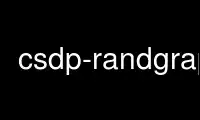# csdp-randgraph - Online in the CloudThis is the command csdp-randgraph that can be run in the OnWorks free hosting provider using one of our multiple free online workstations such as Ubuntu Online, Fedora Online, Windows online emulator or MAC OS online emulator

### PROGRAM:

#### NAME

csdp - semidefinite program solver

#### SYNOPSIS

csdp <problemfile> <finalsolution> <initialsolution>
csdp-complement <inputgraph> <outputgraph>
csdp-graphtoprob <graph> <problemfile>
csdp-randgraph <rand_graph> <file> <n> <p> [<seed>]
csdp-theta <graph>

#### DESCRIPTION

This manual page documents briefly the csdp, csdp-complement, csdp-graphtoprob, csdp-
randgraph and csdp-theta commands.

csdp -- interface to solve general semi-definite programs
csdp-complement -- compute the complement of a graph and output it in csdp problem format
csdp-graphtoprob -- convert graph into csdp problem format file
csdp-randgraph -- generate a random graph
csdp-theta -- solves the Lovasz thetha problem

#### OPTIONS

A summary of options is included below. For a complete description, see
/usr/share/doc/coinor-csdp-doc/csdpuser.pdf.

csdp
inputproblem in the SDPA sparse format

problemfile
is the name of a file containing the SDP problem in SDPA sparse format

finalsolution
is the optional name of a file in which to save the final solution

initialsolution
is the optional name of a file from which to take the initial solution.

CSDP searches for a file named param.csdp in the current directory. If no such file
exists, then default values for all of CSDP’s parameters are used. If there is a parameter
file, then CSDP reads the parameter values from this file. The default parameter values
is given below (can be pasted into a file):

axtol=1.0e-8
atytol=1.0e-8
objtol=1.0e-8
pinftol=1.0e8
dinftol=1.0e8
maxiter=100
minstepfrac=0.90
maxstepfrac=0.97
minstepp=1.0e-8
minstepd=1.0e-8
usexzgap=1
tweakgap=0
affine=0
printlevel=1
perturbobj=1
fastmode=0

param.csdp file parameter description

axtol atytol objtol tolerances for primal feasibility, dual feasibility, and relative
duality gap

pinftol
dinftol tolerances used in determining primal and dual infeasibility

maxiter
plimit the total number of iterations that CSDP may use

minstepfrac
maxstepfrac determine how close to the edge of the feasible region CSDP will step.
If the primal or dual step is shorter than minstepp or minstepd, then CSDP declares
a line search failure. usexzgap If parameter 0, then CSDP will use the objective
function duality gap instead of the tr(XZ) gap

tweakgap
if set to 1, and usexzgap is set to 0, then CSDP will attempt to "fix" negative
duality gaps.

affine If parameter affine is set to 1, then CSDP will take only primal–dual affine steps
and not make use of the barrier term. This can be useful for some problems that do
not have feasible solutions that are strictly in the interior of the cone of
semidefinite ma- trices. printlevel determines how much debugging information is
output. Use printlevel=0 for no output and printlevel=1 for normal output. Higher
values of printlevel will generate more debugging output.

perturbobj
determines whether the objective function will be perturbed to help deal with
problems that have unbounded optimal solution sets. If per- turbobj is 0, then the
objective will not be perturbed. If perturbobj=1, then the objective function will
be perturbed by a default amount. Larger values of perturbobj (e.g. 100.0) increase
the size of the perturbation. This can be helpful in solving some difficult
problems.

fastmode
determines whether or not CSDP will skip certain time consuming operations that
slightly improve the accuracy of the solutions. If fastmode is set to 1, then CSDP
may be somewhat faster, but also somewhat less accurate.

Use csdp-randgraph online using onworks.net services

Free Servers & Workstations